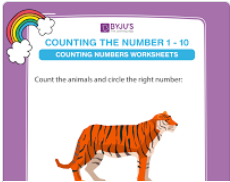# Count & Circle the Correct Number Worksheets

Count and Write numbers is a fundamental exercise for kids. Under this, we have several kinds of worksheets. One of them is Count and Circle the Correct Number Worksheets. In these Count and Write worksheets of Class 1 Maths, kids have to look at the pictures of objects, count and then circle the correct number accordingly. These count and circle the correct number worksheets are helpful in building sound number recognition capabilities in kids.

## Circle the Correct Number Worksheets (Numbers up to 10):